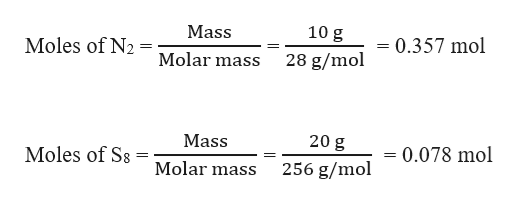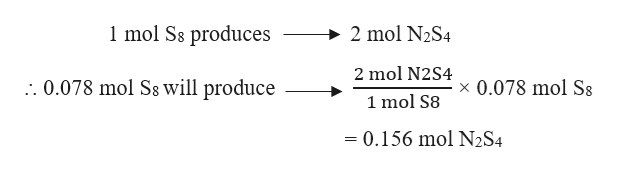# What mass of N2S4is formed when 10.00 g of N2 is heated with 20.0 g S8?

Question
30 views

What mass of N2S4is formed when 10.00 g of Nis heated with 20.0 g S8?

check_circle

Step 1

Given,

Mass of N2 = 10.00 g

Mass of S8 = 20.0 g

The moles of N2 and S8 can be calculated as:help_outlineImage Transcriptionclose10 g Mass 0.357 mol Moles of N2 28 g/mol Molar mass 20 g Mass = 0.078 mol Moles of Ss 256 g/mol Molar mass fullscreen
Step 2

The balanced chemical reaction can be written as:

Step 3

The limiting reagent can be calculated as:

Stochiometric molar ratio (N2 : S8) = 2 : 1

Actual molar ratio (N2 : S8) = 0.357 : 0.078 = 4.5 : 1

Since N2 is in excess amount, so the limiting reagent is S8. T...help_outlineImage Transcriptionclose1 mol Ss produces 2 mol N2S4 2 mol N2S4 . 0.078 mol Ss will produce 0.078 mol Ss X 1 mol S8 = 0.156 mol N2S4 fullscreen

### Want to see the full answer?

See Solution

#### Want to see this answer and more?

Solutions are written by subject experts who are available 24/7. Questions are typically answered within 1 hour.*

See Solution
*Response times may vary by subject and question.
Tagged in

### General Chemistry